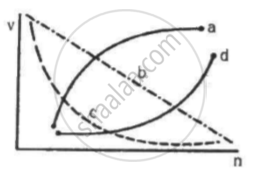Advertisement Remove all ads

# Which of the Following Curves May Represent the Speed of the Electron in a Hydrogen Atom as a Function of the Principal Quantum Number N? - Physics

Short Note

Which of the following curves may represent the speed of the electron in a hydrogen atom as a function of trincipal quantum number n?Advertisement Remove all ads

#### Solution

(c)

The speed (v) of electron can be expressed as

v = (Ze^2)/(2∈_0hn) ....(1)

Here,

Z = Number of protons in the nucleus

e = Magnitude of charge on electron charge

n = Principal quantum number

h = Planck's constant

It can be observed from equation (1) that the velocity of electron is inversely proportional to the principal quantum number (n).
Therefore, the graph between them must be a rectangular hyperbola.
The correct curve is (c).

Concept: The Line Spectra of the Hydrogen Atom
Is there an error in this question or solution?
Advertisement Remove all ads

#### APPEARS IN

HC Verma Class 11, Class 12 Concepts of Physics Vol. 2
Chapter 21 Bohr’s Model and Physics of Atom
MCQ | Q 7 | Page 383
Advertisement Remove all ads
Advertisement Remove all ads
Share
Notifications

View all notifications

Forgot password?